Next: Supersonic Flow in Corner Up: Two-Dimensional Compressible Inviscid Flow Previous: Introduction

# Oblique Shocks

Consider a normal shock in which the flow upstream and downstream of the shock front is parallel to the-axis, and front itself lies in the-plane. (See Section 14.8.) Suppose that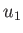and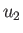are the upstream and downstream flow speeds, respectively. Let us now view this shock in a frame of reference that moves with respect to our original frame at the constant velocity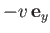. As illustrated in Figure 15.1, viewing the shock in the new reference frame has the effect of adding a component of velocity of magnitude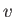, directed parallel to the-axis, to both the upstream and the downstream flow. The resultant upstream velocity is now of magnitude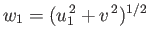, and subtends an angle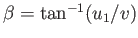, known as the wave angle, with the shock front. It is evident that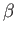may be adjusted to any value via a suitable choice of. Now, becauseis not the same as, the inclination of the downstream flow to the shock front is different to that of the upstream flow. In other words, the direction of the flow turns abruptly as it passes through the shock. Becauseis always less than(see Section 14.8), the deviation is always towards the shock front. In other words, the deflection angle,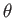, defined in Figure 15.1, is positive.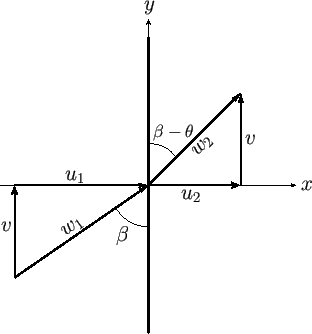The relationship between the conditions upstream and downstream of the shock is easily obtained from the analysis of Section 14.8, because viewing a normal shock in a moving frame of reference does not change the relationship between the upstream and downstream conditions in the original reference frame. The only modification is that the upstream Mach number is now defined as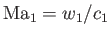, rather than. Here,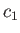is the upstream sound speed. Thus, given that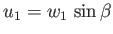, we simply need to make the transformation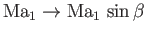in the previous analysis. Hence, Equations (14.107), (14.108), (14.109), and (14.113), yield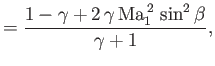(15.1)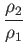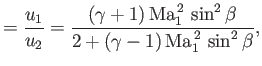(15.2)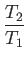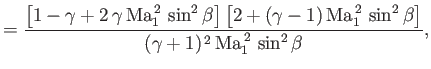(15.3)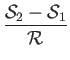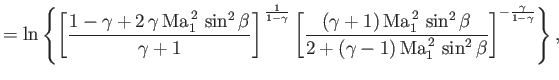(15.4)

respectively. Here,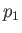,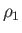,, and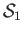are the upstream pressure, density, temperature, and specific entropy, respectively,,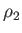,, and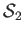are the corresponding downstream quantities,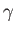is the ratio of specific heats,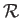the specific gas constant, and use has been made of Equation (14.59). As before (see Section 14.8), the second law of thermodynamics demands that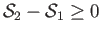, which implies that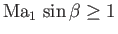. This sets a minimum inclination of the upstream flow to the shock front for a given upstream Mach number. The maximum inclination is, of course,. Thus,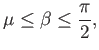(15.5)

where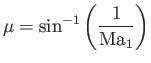(15.6)

is termed the Mach angle. The downstream Mach number can be calculated by noting that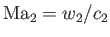, rather that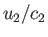, where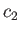is the downstream sound speed, and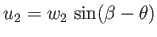. In other words, we simply need to make the transformation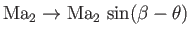in the previous analysis. Thus, it follows from Equation (14.104) that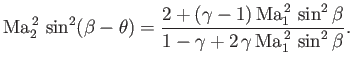(15.7)

According to Figure 15.1,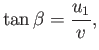(15.8)

and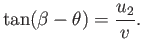(15.9)

Eliminating, and then making use of Equation (15.2), we obtain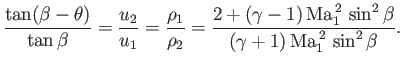(15.10)

Now,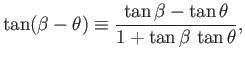(15.11)

so Equation (15.10) can be rearranged to give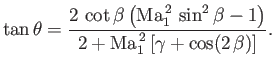(15.12)

Here, use has been made of the identity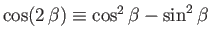. The previous expression implies thatat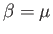and, which are the limits of the range of allowed values fordefined in Equation (15.5). Within this range,is positive, and, must, therefore, have a maximum value,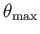. This is illustrated in Figure 15.2, where the relationship betweenand, for an ideal gas with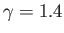, is plotted for various values of.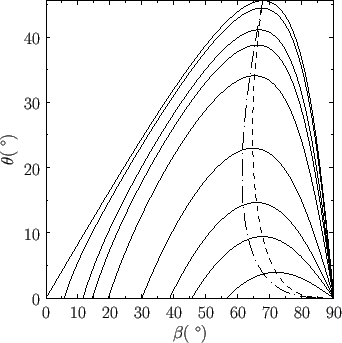If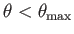then, for each value ofand, there are two possible solutions, corresponding to two different values of. The larger value ofcorresponds to the stronger shock [because, according to Equation (15.1), the shock strength,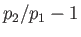, is a monotonically increasing function of].

Also shown in Figure 15.2 is the locus of solutions for which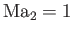. In the solution with the stronger shock, the downstream flow always becomes subsonic. On the other hand, in the solution with the weaker shock, the downstream flow remains supersonic, except for a small range of values ofthat are slightly smaller that.

Equation (15.10) can be rearranged to give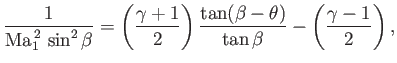(15.13)

which can be further reduced to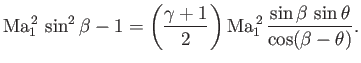(15.14)

For small deflection angles,, the previous expression can be approximated by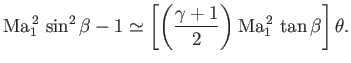(15.15)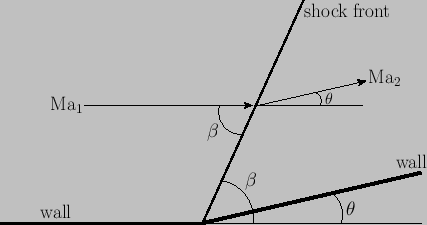Next: Supersonic Flow in Corner Up: Two-Dimensional Compressible Inviscid Flow Previous: Introduction
Richard Fitzpatrick 2016-03-31